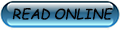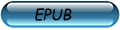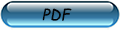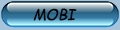###Author Topic: Basics of Algebra, Topology, and Differential Calculus  (Read 262 times)

####Sierra##### Basics of Algebra, Topology, and Differential Calculus
« Reply #1 on: Feb:06:2019 »Author: Jean Gallier /Genre: Mathematics

In the first chapter, the basic algebraic structures (groups, rings, felds, vector spaces) are reviewed, with a major emphasis on vector spaces. Basic notions of linear algebra such as vector spaces, sub spaces, linear combinations, linear independence, bases, quotient spaces, linear maps, matrices, change of bases, direct sums, linear forms, dual spaces, hyper planes, transpose of a linear maps, are reviewed.

Linear Algebra

Determinants

Gaussian Elimination, LU, Cholesky, Echelon Form

Vector Norms and Matrix Norms

Iterative Methods for Solving Linear Systems

Euclidean Spaces

QR-Decomposition for Arbitrary Matrices

Hermitian Spaces

Eigenvectors and Eigenvalues

Spectral Theorems

Bilinear Forms and Their Geometries

Introduction to The Finite Elements Method

Singular Value Decomposition and Polar Form

Applications of SVD and Pseudo-inverses

Basics of Affine Geometry

Polynomials, Ideals and PID’s

UFD’s, Noetherian Rings, Hilbert’s Basis Theorem

Annihilating Polynomials; Primary Decomposition

Tensor Algebras

Introduction to Modules; Modules over a PID

Normal Forms; The Rational Canonical Form

Topology

A Detour On Fractals

Differential Calculus

Extrema of Real-Valued Functions

Newton’s Method and its Generalizations